Next: Parallel Wave Propagation Up: Waves in Cold Plasmas Previous: Waves in an Unmagnetized

# Low-Frequency Wave Propagation

Let us now consider wave propagation through a magnetized plasma at frequencies far below the ion cyclotron or plasma frequencies, which are, in turn, well below the corresponding electron frequencies. In the low-frequency regime (i.e.,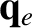), we have [see Eqs. (476)-(478)]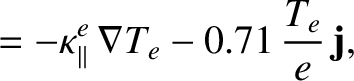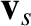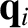(522)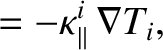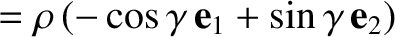(523)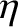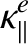(524)

Here, use has been made of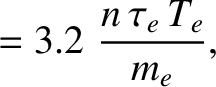. Thus, the eigenmode equation (490) reduces to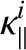(525)

The solubility condition for Eq. (525) yields the dispersion relation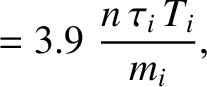(526)

Note that in the low-frequency ordering,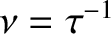. Thus, we can see that the bottom right-hand element of the above determinant is far larger than any of the other elements, so to a good approximation the roots of the dispersion relation are obtained by equating the term multiplying this large factor to zero. In this manner, we obtain two roots:(527)

and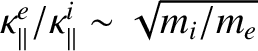(528)

It is fairly easy to show, from the definitions of the plasma and cyclotron frequencies [see Eqs. (465)-(468], that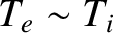(529)

Here,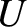is the plasma mass density, and(530)

is called the Alfvén velocity. Thus, the dispersion relations of the two low-frequency waves can be written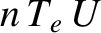(531)

and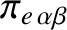(532)

Here, we have made use of the fact that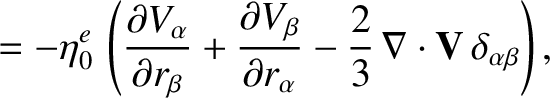in conventional plasmas.

The dispersion relation (531) corresponds to the slow or shear Alfvén wave, whereas the dispersion relation (532) corresponds to the fast or compressional Alfvén wave. The fast/slow terminology simply refers to the ordering of the phase velocities of the two waves. The shear/compressional terminology refers to the velocity fields associated with the waves. In fact, it is clear from Eq. (525) that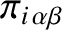for both waves, whereas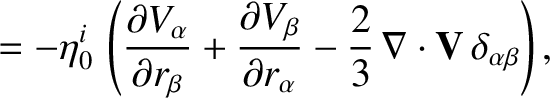for the shear wave, and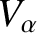for the compressional wave. Both waves are, in fact, MHD modes which satisfy the linearized MHD Ohm's law [see Eq. (387)]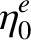(533)

Thus, for the shear wave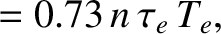(534)

and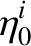, whereas for the compressional wave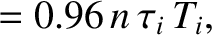(535)

and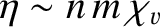. Now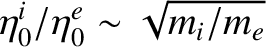. Thus, the shear-Alfvén wave is a torsional wave, with zero divergence of the flow, whereas the compressional wave involves a non-zero divergence of the flow. It is important to realize that the thing which is resisting compression in the compressional wave is the magnetic field, not the plasma, since there is negligible plasma pressure in the cold-plasma approximation.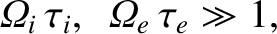Figure 8 shows the characteristic distortion of the magnetic field associated with a shear-Alfvén wave propagating parallel to the equilibrium field. Clearly, this wave bends magnetic field-lines without compressing them. Figure 9 shows the characteristic distortion of the magnetic field associated with a compressional-Alfvén wave propagating perpendicular to the equilibrium field. Clearly, this wave compresses magnetic field-lines without bending them.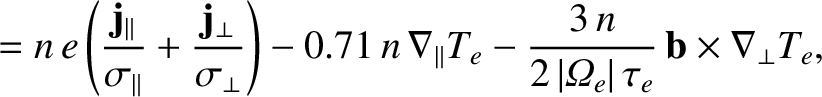It should be noted that the thermal velocity is not necessarily negligible compared to the Alfvén velocity in conventional plasmas. Thus, we can expect the dispersion relations (531) and (532) to undergo considerable modification in a warm'' plasma (see Sect. 5.4).Next: Parallel Wave Propagation Up: Waves in Cold Plasmas Previous: Waves in an Unmagnetized
Richard Fitzpatrick 2011-03-31